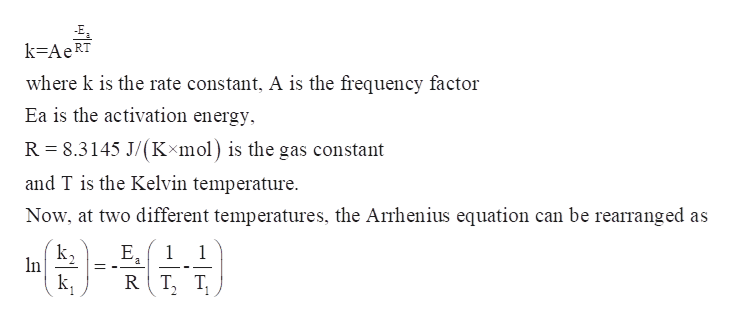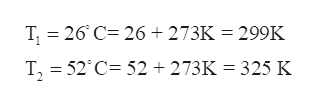To use the Arrhenius equation to calculate the activation energy.As temperature rises, the average kinetic energy of molecules increases. In a chemical reaction, this means that a higher percentage of the molecules possess the required activation energy, and the reaction goes faster. This relationship is shown by the Arrhenius equationk=Ae−Ea/RTwhere k is the rate constant, A is the frequency factor, Ea is the activation energy, R = 8.3145 J/(K⋅mol) is the gas constant, and T is the Kelvin temperature. The following rearranged version of the equation is also useful:ln(k2k1)=(EaR)(1T1−1T2)where k1 is the rate constant at temperature T1, and k2 is the rate constant at temperature T2.The rate constant of a chemical reaction increased from 0.200 s−1 to 2.80 s−1 upon raising the temperature from 26.0 ∘C to 52.0 ∘C .Calculate the value of (1T1−1T2) where T1 is the initial temperature and T2 is the final temperature.Express your answer numerically.

Question
To use the Arrhenius equation to calculate the activation energy.

As temperature rises, the average kinetic energy of molecules increases. In a chemical reaction, this means that a higher percentage of the molecules possess the required activation energy, and the reaction goes faster. This relationship is shown by the Arrhenius equation

k=Ae−Ea/RT

where k is the rate constant, A is the frequency factor, Ea is the activation energy, R = 8.3145 J/(K⋅mol) is the gas constant, and T is the Kelvin temperature. The following rearranged version of the equation is also useful:

ln(k2k1)=(EaR)(1T1−1T2)

where k1 is the rate constant at temperature T1, and k2 is the rate constant at temperature T2.

The rate constant of a chemical reaction increased from 0.200 s−1 to 2.80 s−1 upon raising the temperature from 26.0 ∘C to 52.0 ∘C .

Calculate the value of (1T1−1T2) where T1 is the initial temperature and T2 is the final temperature.
Step 1

The value of (1/T1−1/T2) is to be calculated for the rearranged version of the Arrhenius equation given that-

The rate constant of a chemical reaction increased from 0.200 s−1 to 2.80 s−1 upon raising the temperature from 26.0 ∘C to 52.0 ∘C.

Step 2

Arrhenius equation relates the rate constants to the temperature.help_outlineImage TranscriptioncloseE k-AeRT where k is the rate constant, A is the frequency factor Ea is the activation energy R 8.3145 J/(Kxmol) is the gas constant and T is the Kelvin temperature Now, at two different temperatures, the Arrhenius equation can be rearranged as E Е, 1 a 1 In k RT T fullscreen
Step 3

Convert the given temperature’s unit into kelvin -

Given –

T1 = 26°C

T2 = 52°C

...help_outlineImage TranscriptioncloseT 26 C 26 273K = 299K T, 52 C 52 273K 325 K fullscreen

Want to see the full answer?

See Solution

Want to see this answer and more?

Our solutions are written by experts, many with advanced degrees, and available 24/7

See Solution
Tagged in

General Chemistry